Good habits: aggregate and optimize
Share
Explore
Aggregate common data points
Approach
Create a summary table aggregating the data from the larger table.
In the case of our sample data setup, and what is true for most tables of data, we have repeated values. The total number of countries is far fewer than the total number of rows, and most of our data needs to be cut by country.
Here’s where we can create a second table listing each country once and allow the full data table to reference these values instead of repeating these values. In Coda we call this a lookup.
The table is the same data set we worked with in the first strategy. Since there are 185 unique countries, which is far fewer than the 1,000 total rows, we’re going to pull these out into their own . Then we can set the Country column in the data table to lookup to the new table.
This can actually be done in one step in Coda. In the column menu, choose
Column type → Lookup → Create new table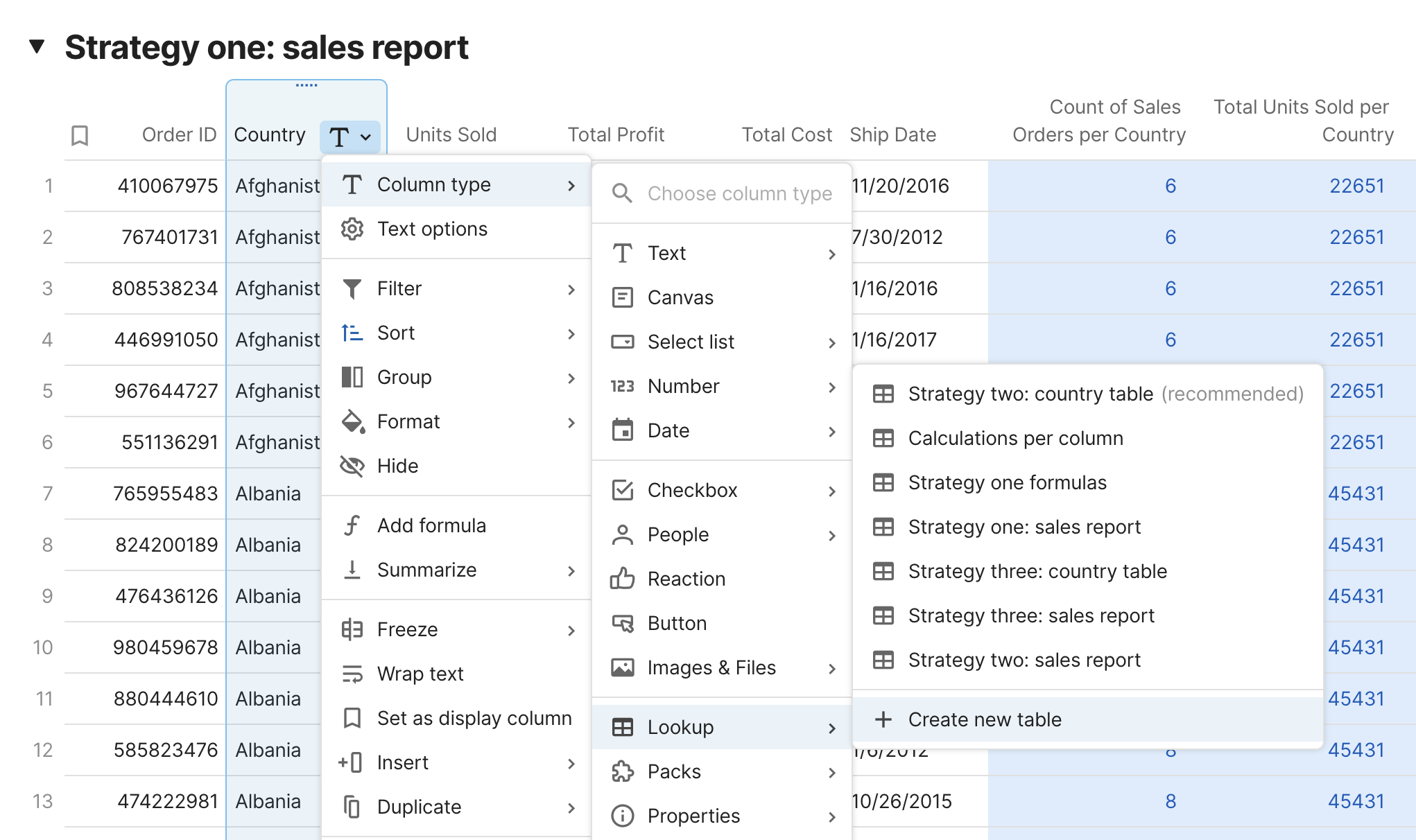These two tables are now connected and we can start writing formulas for our specific data points. We still need to look to the full data table, but since there are only 185 rows in the country table, we only need to go through this process 185 times.
185 rows in the country table multiplied by 1,000 rows in the data table equals 185,000 estimated calculations.
Strategy two: country table =
185
rows
Strategy two: sales report =
1000
rows
Search
1
Count of Sales Orders per Country
Calculations: 185,000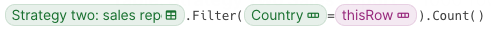2
Total Units Sold per Country
Calculations: 185,000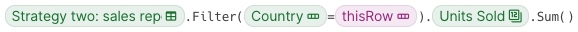3
Total Profit per Country
Calculations: 185,000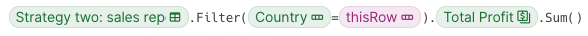4
Total Cost per Country
Calculations: 185,000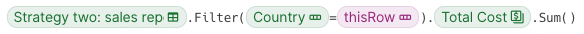5
Most Recent Ship Date per Country
Calculations: 185,000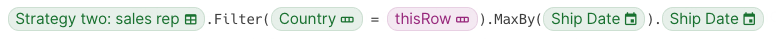There are no rows in this table
Total calculations for five data points: 925,000
This is a difference of 5x and that difference will continue to grow as more data points are added. This is not only great news for processing calculations, but our view of the aggregate data has also been simplified.
We can now sort and filter this new summary table much more easily and we no longer have to wrestle with charts either.
Can we optimize this even further?
Let’s check out strategy three
Strategy two: country table
0
Strategy two: sales report
0